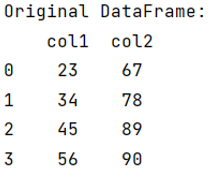# How to create a dataframe while preserving order of the columns?

Learn, how can we create a dataframe while preserving order of the columns?
Submitted by Pranit Sharma, on September 12, 2022

Pandas is a special tool that allows us to perform complex manipulations of data effectively and efficiently. Inside pandas, we mostly deal with a dataset in the form of DataFrame. DataFrames are 2-dimensional data structures in pandas. DataFrames consist of rows, columns, and data.

## Creating a dataframe while preserving order of the columns

We are supposed to create a DataFrame with multiple NumPy arrays or pandas Series while preserving the order of each item, we will pass the key-value tuple pair for order preservation. We will use the OrderedDict() method which is a method of collections library. We will pass the key-value pair inside OrderedDict(). Since while constructing a dictionary, the order is randomized, OrderedDict() will work accordingly if we pass a key-value tuple pair.

Let us understand with the help of an example,

## Python code to create a dataframe while preserving order of the columns

```# Importing pandas package
import pandas as pd

# Importing numpy package
import numpy as np

# Importing orderdict method
# from collections
from collections import OrderedDict

# Creating numpy arrays
arr1 = np.array([23,34,45,56])
arr2 = np.array([67,78,89,90])

# Creating DataFrame
df = pd.DataFrame(OrderedDict({'col1':pd.Series(arr1),'col2':pd.Series(arr2)}))

# Display the DataFrame
print("Original DataFrame:\n",df,"\n")
```

Output:What's New (MCQs)

Top Interview Coding Problems/Challenges!

Languages: » C » C++ » C++ STL » Java » Data Structure » C#.Net » Android » Kotlin » SQL
Web Technologies: » PHP » Python » JavaScript » CSS » Ajax » Node.js » Web programming/HTML
Solved programs: » C » C++ » DS » Java » C#
Aptitude que. & ans.: » C » C++ » Java » DBMS
Interview que. & ans.: » C » Embedded C » Java » SEO » HR
CS Subjects: » CS Basics » O.S. » Networks » DBMS » Embedded Systems » Cloud Computing
» Machine learning » CS Organizations » Linux » DOS
More: » Articles » Puzzles » News/Updates

© https://www.includehelp.com some rights reserved.